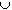#Interactive Real Analysis

Next | Previous | Glossary | Map

## 5. Topology

### 5.3. Connected and Disconnected Sets

In the last two section we have classified the open sets, and looked at two classes of closed set: the compact and the perfect sets. In this section we will introduce two other classes of sets: connected and disconnected sets.
 Definition 5.3.1: Connected and Disconnected An open set S is called disconnected if there are two open, non-empty sets U and V such that: UV = 0 UV = S A set S (not necessarily open) is called disconnected if there are two open sets U and V such that (US) # 0 and (VS) # 0 (US)(VS) = 0 (US)(VS) = S If S is not disconnected it is called connected.
Note that the definition of disconnected set is easier for an open set S. In principle, however, the idea is the same: If a set S can be separated into two open, disjoint sets in such a way that neither set is empty and both sets combined give the original set S, then S is called disconnected.

To show that a set is disconnected is generally easier than showing connectedness: if you can find a point that is not in the set S, then that point can often be used to 'disconnect' your set into two new open sets with the above properties.

 Examples 5.3.2:Is the set { xR : | x | < 1, x # 0 } connected or disconnected ? What about the set { xR : | x |1, x # 0 }Is the set [-1, 1] connected or disconnected ?Is the set of rational numbers connected or disconnected ? How about the irrationals ?Is the Cantor set connected or disconnected ?
In the real line connected set have a particularly nice description:
 Proposition 5.3.3: Connected Sets in R are Intervals If S is any connected subset of R then S must be some interval.

Hence, as with open and closed sets, one of these two groups of sets are easy:

• open sets in R are the union of disjoint open intervals
• connected sets in R are intervals
The other group is the complicated one:
• closed sets are more difficult than open sets (e.g. Cantor set)
• disconnected sets are more difficult than connected ones (e.g. Cantor set)
In fact, a set can be disconnected at every point.
 Definition 5.3.4: Totally Disconnected A set S is called totally disconnected if for each distinct x, yS there exist disjoint open set U and V such that xU, yV, and (US)(VS) = S.
Intuitively, totally disconnected means that a set can be be broken up into two pieces at each of its points, and the breakpoint is always 'in between' the original set.
 Example 5.3.5:The Cantor set is disconnected. Is it totally disconnected ?Is the set {0, 1} connected or disconnected ? Is it totally disconnected ?Is the set {1, 1/2, 1/3, 1/4, ...} totally disconnected ? How about the set {1, 1/2, 1/3, 1/4 ...}{0} ?Find a totally disconnected subset of the interval [0, 1] of length 0 (different from the Cantor set), and another one of length 1.
Next | Previous | Glossary | Map